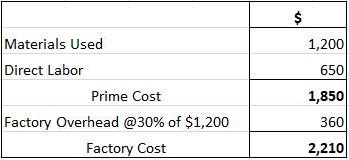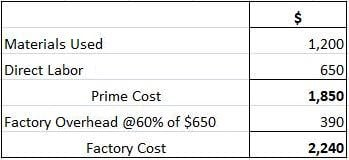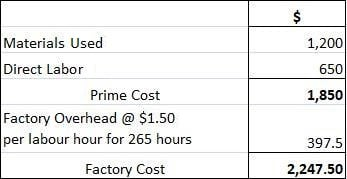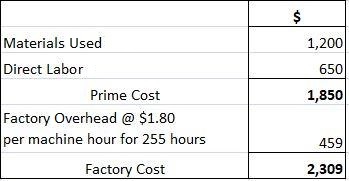These methods apply to absorbing the factory overhead:

## (1) Units of Output Method

Absorption of the factory overhead occurs based on the number of units manufactured. Dividing the overhead expenses by the number of units of output obtains the overhead rate.

### Formula

Overhead rate = factory overhead of the department / number of units of output.

### Example

If the factory overhead for department ‘A’ for a particular period amounts to \$10,000 and the department manufactures 2,000 units, the overhead rate per unit amounts to \$5 (\$10,000 + 2,000 units). Each unit will bear \$5 by way of the overhead cost.

This simple, direct method only applies to single output industries manufacturing one product or a few grades of the same product.

## (2) Direct Material Cost Method (Percentage on Direct Material Cost)

Here, the overhead absorption occurs based on the direct materials consumed. This method expresses the overhead rate as a percentage of the direct material cost.

Calculate the overhead rate by dividing the factory overhead for a particular period by the cost of the direct materials used in the same period and then multiplying it by 100.

### Formula

Overhead rate = (factory overhead cost of direct materials used) x 100.

### Example

If the monthly amount or factory overhead for department ‘A’ amounts to \$10,000 and the cost of direct material consumed amounts to \$25,000, the overhead rate is 40% on the direct material cost (10,000 / 25,000) x 100.

A job carried out by the department involving direct material costing \$200 means the job absorbs \$80 (40% of \$200) and Rs. (40% 01′ Rs. 2()()) by way of overhead.

This simple, equitable method uses the same type of material in all the manufactured articles, and the price of such material does not respond to fluctuations.

1. Considerable fluctuations, unaccompanied by similar fluctuations in the factory overhead, affect the cost of materials and render this basis unstable.
2. Ignores the time factor involved in production.
3. A job’s consumption of expensive materials does not justify supposing that charging the factory overhead to it would be proportionately higher.
4. Factory expenses result from the cost of materials and the type of workers. However, this method makes no distinction between skilled and unskilled workers.

## (3) Direct Labor Cost Method (Percentage on Direct Wages)

Under this method, the overhead absorption occurs based on direct wages.

Obtain the overhead rate, expressed as a percentage on direct wages, by dividing the factory overhead for a particular period by the direct wages paid during that period and multiplying it by 100.

### Formula

Overhead rate = (factory overhead / direct wages) x 100.

### Example

If the factory overhead cost for department ‘A’ for a particular month amounts to \$10,000 and the total labor cost amounts to \$20,000, the overhead rate will be 50% on direct wages (10,000 / 20,000) x 100.

A job carried out by department ‘A’ involving a payment of \$200 by way of direct wages absorbs \$100 (50% of \$200) by way of overhead.

1. Automatic consideration of the time factor because wages are paid based on time.
2. Wage rates show more constancy than material prices, meaning this method provides a stable basis for overhead absorption.
3. Factory expenses can depend on the number of employees, making this basis more equitable.

1. No consideration of skilled and unskilled workers’ wage rates.
2. No distinction between the efforts of hand workers and machine workers.
3. No consideration of the time element if the workers receive payment based on piece work and not the basis of time.

## (4) Prime Cost Method (Percentage on Prime Cost)

This method absorbs the overhead based on the prime cost and assumes that both materials and labor give rise to the overhead. The overhead rate is expressed as a percentage of the prime cost.

### Formula

Overhead rate = (factory overhead / prime cost) x 100.

### Example

If the factory overhead for department ‘A’ for a particular month amounts to \$10,000 and the prime cost amounts to \$40,000, the overhead rate will be 25% on the prime cost (10,000 / 40,000) x 100.

If the prime cost of a job done by the department amounts to \$300, the job will absorb \$75 (25% of \$300) by way of overhead.

This simple method assigns equal weight to materials and labor.

## (5) Direct Labor Hour Rate Method

Absorption of the overhead reflects the number of hours involving direct labor.

Calculate the direct labor hour rate of the overhead by dividing the factory overhead for a given period by the total number of direct labor hours for the same period.

### Formula

Overhead rate = factory overhead / number of direct labor hours.

### Example

If the factory expenses for department ‘A’ for a particular period amount to \$10,000 and the direct labor hours during the same period amount to 5,000, the direct labor hour rate will be \$2 (\$10,000 / 5,000 hours).

A particular job carried out during the period involving 50 hours of direct labor absorbs \$100 (50 hours x \$2) by way of overhead.

The direct labor hour rate is a time rate. Most factory overhead items vary with time, meaning this method provides a basis for overhead absorption.

This method also assumes the predominance of direct labor and ignores all other production factors.

## (6) Machine Hour Rate Method

Overhead absorption occurs based on the cost of operating machinery. Highly organized engineering works relying heavily on machines tend to apply this method.

The hourly cost of operating a machine, otherwise known as the machine hour rate, represents the overhead rate.

Obtain the machine hour rate by dividing the factory expenses connected with a machine for a given period by the number of hours worked by the machine during that period.

### Formula

Overhead rate = factory overhead (relating to a machine) / number of machine hours.

### Example

If the factory expenses relating to Machine No. 15 installed in department ‘A’ for a particular period amount to \$5,000 and said machine works 1,000 hours during the period, the machine hour rate amounts to \$5 (\$5,000 + 1,000 hours).

A particular job executed by Machine No. 15 taking 30 hours absorbs \$150 (30 hours x \$5) by way of the factory overhead.

In mechanized factories, the machine hour rate method provides the most scientific basis for absorbing the overhead.

However, this complicated method involves too many mathematical calculations and requires accurate records of the machines and repairs and maintenance costs.

## (7) Dual Hour Rate Method

This method combines the direct labor rate and the machine hour rate. It classifies the overheads into two categories related to manual work and machine work.

The labor hour rate absorbs the first category of overheads and the machine hour rate absorbs the second category of overheads.

### Illustration

The following information relates to a Manufacturing Co., for the year ending 31 March 2019:

• Materials used \$60,000.
• Direct labor \$30,000.
• Factory overhead \$18,000.
• Direct labor hours 12,000.
• Machine hours 10,000.
• The following information relates to Job No. 75:
• Materials used \$1,200.
• Direct labor \$650.
• Direct labor hours 265.
• Machine hours 255.

Enumerate four different methods of absorbing factory overheads and prepare a statement showing the total cost of Job No. 75 under each of the four methods referred to above.

### Solution

The four primary methods of absorbing the factory overhead may involve:

1. A percentage of direct materials.
2. A percentage of the direct labor cost.
3. The direct labor hour rate method.
4. The machine hour rate method.

### First Method

The overhead rate as a percentage on the direct materials cost comes to 30%, calculated as follows:

= (18,000 / 60,000) x 100.

The factory cost of the job will be as follows:

###Second Method

The overhead rate as a percentage on direct labor cost comes to 60%, calculated as follows:

= (18,000 / 30,000) x 100.

The factory cost of the job will be as follows:

###Third Method

The direct labor hour rate of the overhead comes to \$1.50, calculated as follows:

= (\$18,000 / 12,000 hours).

The factory cost of the job will be as follows:

###Fourth Method

The machine hour rate of the overhead comes to \$1.80, calculated as follows:

= \$18,000 / 10,000 hours.

The factory cost of the job will be as follows:## Choice of Overhead Absorption Method

Different methods can absorb the factory overhead: direct material cost, direct labor cost, direct labor hour rate, and machine hour rate. Failing to choose the most equitable and suitable method can result in misleading costing results.

Consider the following factors when selecting a method:

1. The type of industry — whether job order or continuous production.
2. Nature of the manufacturing process — whether manual or mechanical.
3. The primary constituents of the overhead and their nature — whether fixed or variable.
4. The organizational setup of the undertaking into departments and cost centers.
5. Capacity at which the factory works — full-capacity or under-capacity.
6. Management policy.

## Requisites of a Good Method of Absorption

1. A simple, convenient application that does not require unnecessary clerical records.
2. It ensures the recovery of the overhead which is more or less equal to the actual amount of the overhead.
3. It considers the time factor involved in production.
4. It distinguishes between the work of skilled workers and unskilled workers.
5. It makes a proper distinction between manual labor and machine labor.
6. It should prefer multiple rates (determined in relation to the work of different departments) rather than the blanket rate or the overall overhead rate of the entire factory.

## Frequently Asked Questions

### What is a factory overhead?

Factory overhead or factory burden refers to all the costs involved in operating an organization's factory, apart from direct materials and direct labor. In other words, it means indirect cost. Factory overhead may include ,but not limited to: indirect material costs such as lubricants, cleaning materials used for production line, etc. Indirect labor costs such as supervisors, quality control personnel, etc. Factory rent or lease, utilities (electricity, water), Depreciation on factory building and equipment, insurance, taxes.

### What is the purpose of absorption of factory overhead?

The main purpose of absorption of factory overheads is to allocate all the indirect costs of running a factory to individual products or services. This is done in order to determine the cost of each product or service and thereby ascertain whether a business is making profits or losses on specific items.

### What are the different methods of absorption of factory overhead?

There are four common methods of absorption of factory overheads: percentage of direct materials cost, percentage of direct labor cost, direct labor hour rate and machine hour rate.

### How is the overhead rate calculated in the first method?

The overhead rate as a percentage on the direct materials cost comes to 30%, calculated as follows: = (18,000 / 60,000) x 100.

### How is the overhead rate calculated in the first method?

The overhead rate as a percentage on the direct labor cost comes to 18%, calculated as follows: = (18,000 / 100,000) x 100.

True is a Certified Educator in Personal Finance (CEPF®), a member of the Society for Advancing Business Editing and Writing, contributes to his financial education site, Finance Strategists, and has spoken to various financial communities such as the CFA Institute, as well as university students like his Alma mater, Biola University, where he received a bachelor of science in business and data analytics.

To learn more about True, visit his personal website, view his author profile on Amazon, his interview on CBS, or check out his speaker profile on the CFA Institute website.